Latest Banking jobs   »   Quantitative Aptitude Quiz For IBPS Clerk...

# Quantitative Aptitude Quiz For IBPS Clerk Prelims 2021- 13th November

Directions (1 -5): The following questions are accompanied by two statements (I) and (II). You have to determine which statements(s) is/are sufficient/necessary to answer the questions.
(a) Statement (I) alone is sufficient to answer the question but statement (II) alone is not sufficient to answer the questions.
(b) Statement (II) alone is sufficient to answer the question but statement (I) alone is not sufficient to answer the question.
(c) Both the statements taken together are necessary to answer the questions, but neither of the statements alone is sufficient to answer the question.
(d) Either statement (I) or statement (II) by itself is sufficient to answer the question.
(e) Statements (I) and (II) taken together are not sufficient to answer the question.

Q1. In a test there are three section Quant, Reasoning & English. Find the marks obtained by Veer in quant.
I. Average number of marks obtain by Veer in Reasoning & English is 26.
II . And total marks obtain by Veer in Quant & Reasoning is 30.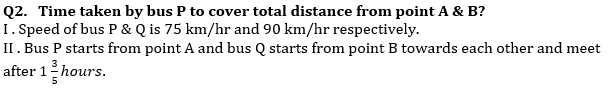Q3. Find the area of circle.
I . Sum of circumference and diameter of circle is equal to perimeter of square, whose side is 29 cm.
II . Difference between circumference and diameter of circle is equal to perimeter of square, whose side is 15 cm.

Q4. Cost price of a jeans is 70% of its marked price. What is the discount% given on the marked price of the jeans?
I . Profit earned by shopkeeper on selling the item at Rs. 2400 is Rs. 300.
II . Cost price of the item is Rs. 2100.

Q5. A boat X is travelling upstream with speed half of its speed in still water. Find distance covered by boat Y travelling upstream in 5 hours.
I . Sum of speed of boat X & Y in still water is 100 km/hr.
II . In downstream, Boat X cover 40 km less distance than Y in two hours.

Directions (6-10): In the given questions, two quantities are given, one as ‘Quantity I’ and another as ‘Quantity II’. You must determine relationship between two quantities and choose the appropriate option.

Q6. Two cards are drawn randomly from a pack of 52 cards.
Quantity I: Find probability of both drawn cards being red color cards.
Quantity II: Find probability of both drawn cards being either heart cards or diamond cards.
(a) Quantity I > Quantity II
(b) Quantity I < Quantity II
(c) Quantity I ≥ Quantity II
(d) Quantity I ≤ Quantity II
(e) Quantity I = Quantity II or no relation.

Q7. Two squares are formed in such a way that square A is larger square and square B is formed by joining midpoints of sides of square A.
Quantity I – area of a square whose side is equal to diagonal of square B.
Quantity II – area of an equilateral triangle whose side is equal to diagonal of square A.
(a) Quantity I > Quantity II
(b) Quantity I ≥ Quantity II
(c) Quantity I ≤ Quantity II
(d) Quantity I < Quantity II
(e) Quantity I = Quantity II or no relation.

Q8. Radius of conical tent and spherical ball is same, and ratio of radius and height of conical tent is 1 : 4.
Quantity I: Volume of conical tent.
Quantity II: Volume of spherical ball.
(a) Quantity I > Quantity II
(b) Quantity I < Quantity II
(c) Quantity I ≥ Quantity II
(d) Quantity I ≤ Quantity II
(e) Quantity I = Quantity II or no relation.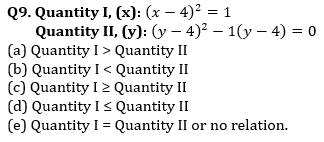Q10. There are 4 numbers – ‘A’, ‘B’, ‘C’ & ‘D’. ‘C’ is 40% more than ‘A’ and ‘D’ is twice of ‘C’. ‘B’ is 1 more than 90% of ‘D’. Average of ‘A’, ‘B’, ‘C’ & ‘D’ is 48.5.
Quantity I: (B + C)
Quantity II: (A + D)
(a) Quantity I > Quantity II
(b) Quantity I < Quantity II
(c) Quantity I ≥ Quantity II
(d) Quantity I ≤ Quantity II
(e) Quantity I = Quantity II or no relation.

Directions (11 -15): In each of these questions, two equations (I) and (II) are given. You have to solve both the equations and give answer
(a) if x>y
(b) if x≥y
(c) if x<y
(d) if x ≤y
(e) if x = y or no relation can be established between x and y.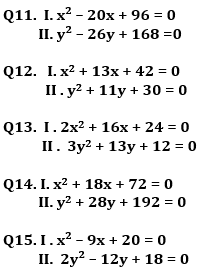Solutions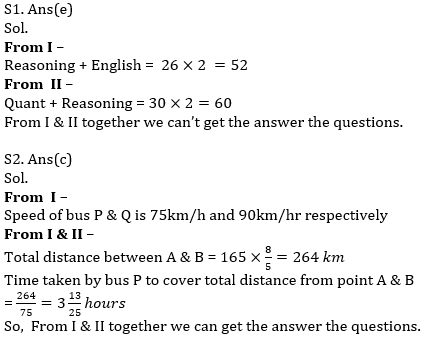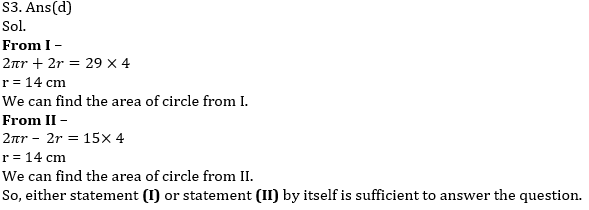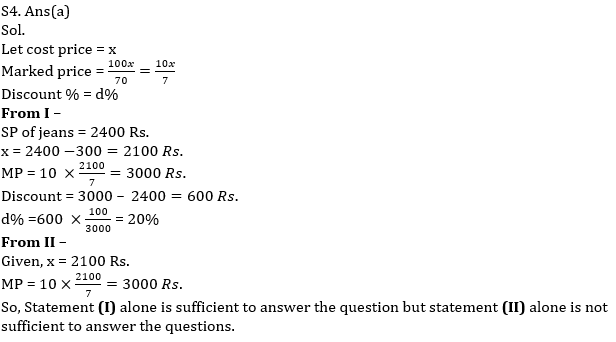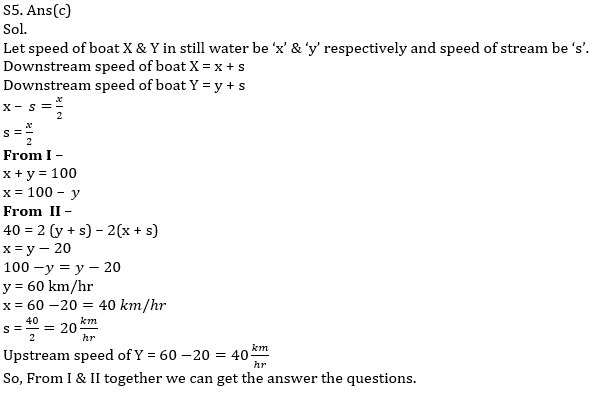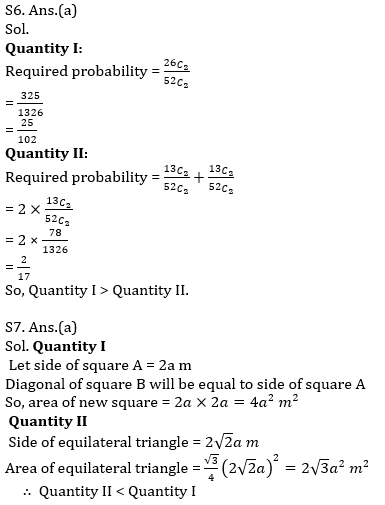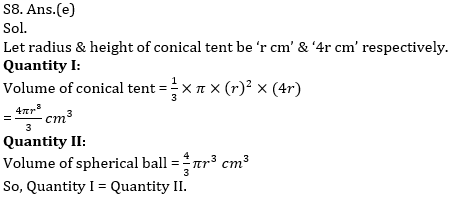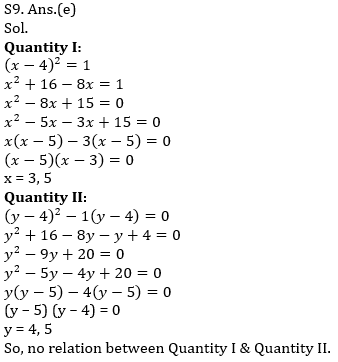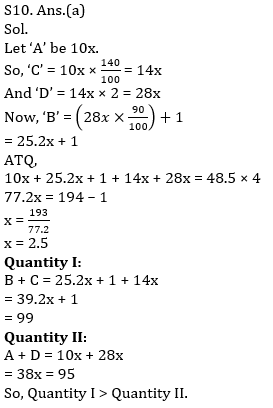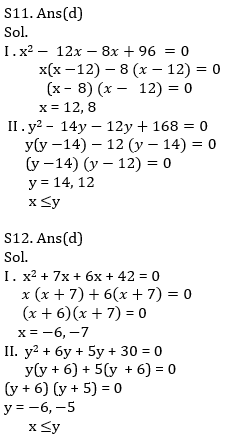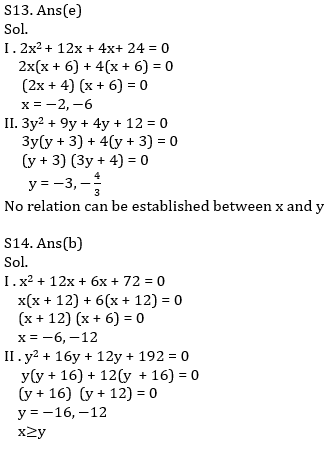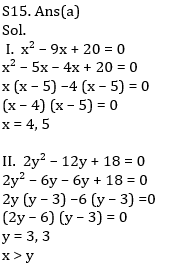×

Thank You, Your details have been submitted we will get back to you.Join India's largest learning destination

What You Will get ?

•Job Alerts
•Daily Quizzes
•Subject-Wise Quizzes
•Current Affairs
•Previous year question papers
•Doubt Solving session

ORJoin India's largest learning destination

What You Will get ?

•Job Alerts
•Daily Quizzes
•Subject-Wise Quizzes
•Current Affairs
•Previous year question papers
•Doubt Solving session

ORJoin India's largest learning destination

What You Will get ?

•Job Alerts
•Daily Quizzes
•Subject-Wise Quizzes
•Current Affairs
•Previous year question papers
•Doubt Solving session

Enter the email address associated with your account, and we'll email you an OTP to verify it's you.Join India's largest learning destination

What You Will get ?

•Job Alerts
•Daily Quizzes
•Subject-Wise Quizzes
•Current Affairs
•Previous year question papers
•Doubt Solving session

Enter OTP

Please enter the OTP sent to
/6

Did not recive OTP?

Resend in 60sJoin India's largest learning destination

What You Will get ?

•Job Alerts
•Daily Quizzes
•Subject-Wise Quizzes
•Current Affairs
•Previous year question papers
•Doubt Solving sessionJoin India's largest learning destination

What You Will get ?

•Job Alerts
•Daily Quizzes
•Subject-Wise Quizzes
•Current Affairs
•Previous year question papers
•Doubt Solving session

Almost there

+91Join India's largest learning destination

What You Will get ?

•Job Alerts
•Daily Quizzes
•Subject-Wise Quizzes
•Current Affairs
•Previous year question papers
•Doubt Solving session

Enter OTP

Please enter the OTP sent to Edit Number

Did not recive OTP?

Resend 60

By skipping this step you will not recieve any free content avalaible on adda247, also you will miss onto notification and job alerts

Are you sure you want to skip this step?

By skipping this step you will not recieve any free content avalaible on adda247, also you will miss onto notification and job alerts

Are you sure you want to skip this step?